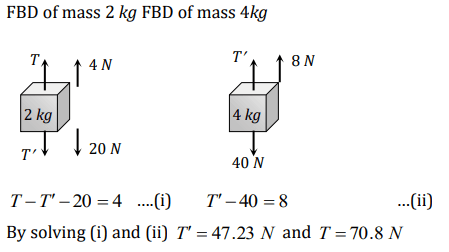## Laws of Motion Questions and Answers Part-17

1. Two forces of magnitude F have a resultant of the same magnitude F. The angle between the two forces is
a) $45^{\circ}$
b) $120^{\circ}$
c) $150^{\circ}$
d) $60^{\circ}$

Explanation: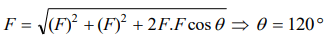2. Two forces with equal magnitudes F act on a body and the magnitude of the resultant force is F/3.The angle between the two forces is
a) $\cos^{-1}-\frac{17}{18}$
b) $\cos^{-1}-\frac{1}{3}$
c) $\cos^{-1}\frac{1}{3}$
d) $\cos^{-1}\frac{8}{9}$

Explanation: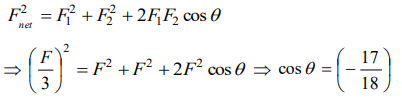3. An object is subjected to a force in the north-east direction. To balance this force, a second force should be applied in the direction
a) North-East
b) South
c) South-West
d) West

Explanation: Direction of second force should be at 180°

4. The resultant force of 5 N and 10 N can not be
a) 12 N
b) 8 N
c) 4 N
d) 5 N

Explanation: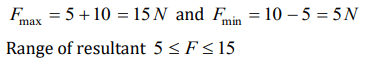5. The resultant of two forces 3P and 2P is R. If the first force is doubled then the resultant is also doubled. The angle between the two forces is
a) $60^{\circ}$
b) $120^{\circ}$
c) $70^{\circ}$
d) $180^{\circ}$

Explanation: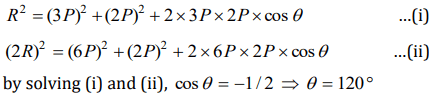6. The resultant of two forces, one double the other in magnitude, is perpendicular to the smaller of the two forces. The angle between the two forces is
a) $60^{\circ}$
b) $120^{\circ}$
c) $150^{\circ}$
d) $90^{\circ}$

Explanation:7. Two forces are such that the sum of their magnitudes is 18 N and their resultant is perpendicular to the smaller force and magnitude of resultant is 12 N. Then the magnitudes of the forces are
a) 12 N, 6 N
b) 13 N, 5 N
c) 10 N, 8 N
d) 16 N, 2 N

Explanation:8. Three forces starts acting simultaneously on a particle moving with velocity $\vec{v}$ . These forces are represented in magnitude and direction by the three sides of a triangle ABC (as shown). The particle will now move with velocity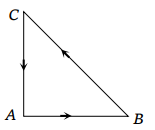a) $\vec{V}$ remaining unchanged
b) Less than $\vec{V}$
c) Greater than $\vec{V}$
d) $\vec{V}$ in the direction of the largest force BC

Explanation: Net force on the particle is zero so the $\vec{v}$ remains unchanged.

9. Which of the following groups of forces could be in equibrium
a) 3 N, 4 N, 5 N
b) 4N, 5 N, 10 N
c) 30N, 40 N, 80 N
d) 1N, 3 N, 5 N

10. Two blocks are connected by a string as shown in the diagram. The upper block is hung by another string. A force F applied on the upper string produces an acceleration of $2 m\diagup s^{2}$  in the upward direction in both the blocks. If T and $T'$ be the tensions in the two parts of the string, thena) $T= 70.8N$   and $T' = 47.2N$
b) $T= 58.8N$    and $T' = 47.2N$
c) $T= 70.8N$   and $T' = 58.8N$
d) $T= 70.8N$   and $T' = 0$